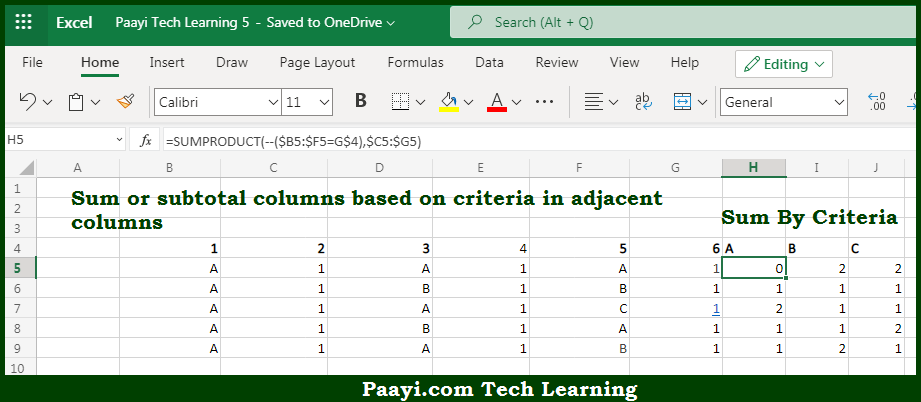# Learn How to SUM Columns Based on Adjacent Criteria in Microsoft Excel

Written by | 0 Comments | 613 Views

In this article, you will learn how to COUNT various things in Microsoft Excel using a single/combination(s) of functions. You will also know how to SUM Columns Based on Adjacent Criteria and see the generic formula.

SUM Columns Based on Adjacent Criteria in Microsoft Excel

The main purpose of this formula is to sum or subtotal columns based on criteria in adjacent columns. Here we will learn how to sum columns based on adjacent criteria. That implies, with the help of a formula based on the SUMPRODUCT function you can sum or subtotal columns based on criteria in adjacent columns. So, with the help of this formula, you can able to sum columns based on adjacent criteria.

General Formula to SUM Columns Based on Adjacent Criteria

=SUMPRODUCT(--(range1=criteria),range2)

The Explanation for the SUM Columns Based on Adjacent CriteriaSo we know that with the help of the given formula above you can able to sum or subtotal columns based on criteria in adjacent columns. Here we will learn how to sum columns based on adjacent criteria. As we know that we are using the SUMPRODUCT function to multiply the sum products of two arrays. The first array, array1 is set up to act as a "filter" to allow only values that meet criteria. Array1 uses a range that begins on the first column that contains values that must pass criteria. The criteria are applied as a test that creates an array of TRUE and FALSE values. So, with the help of this formula, you can able to sum or subtotal columns based on criteria in adjacent columns.